# Subtracting decimals worksheet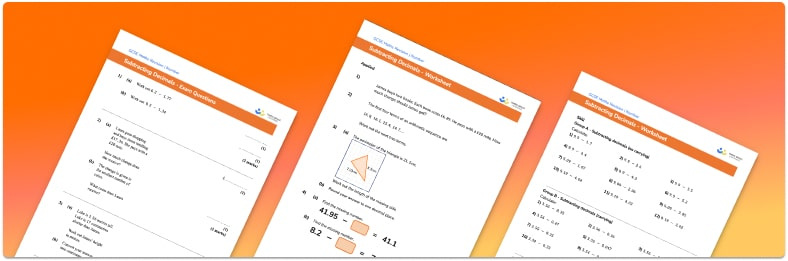• Section 1 of the subtracting decimals worksheet contains 20+ maths skills-based subtracting decimals questions, in 3 groups to support differentiation
• Section 2 contains 3 applied subtracting decimals questions with a mix of real life word problems and deeper problem solving questions
• Section 3 contains 3 foundation and higher level GCSE exam style subtracting decimals questions
• Answers and a mark scheme for all subtracting decimals questions are provided
• Questions follow variation theory with plenty of opportunities for students to work independently at their own level
• All questions created by fully qualified expert secondary maths teachers
• Suitable for GCSE maths revision for AQA, OCR and Edexcel exam boards

• This field is for validation purposes and should be left unchanged.

You can unsubscribe at any time (each email we send will contain an easy way to unsubscribe). To find out more about how we use your data, see our privacy policy.

### Subtracting decimals at a glance

Decimal numbers can be subtracted in the same way as integers. The important thing to consider with subtraction of decimals is place value. Tenths should be subtracted from tenths, hundredths from hundredths, thousandths from thousandths etc.

When using column subtraction to subtract decimals, the key is to ensure the decimal points are lined up. The values in each column can then be subtracted in the same way that we work with whole numbers.

A common application of decimal addition and subtraction is money.

Looking forward, students can then progress to additional decimals worksheets and other number worksheets, for example a multiplying and dividing decimals worksheet or an order of operations worksheet.For more teaching and learning support on Number our GCSE maths lessons provide step by step support for all GCSE maths concepts.

## Related worksheets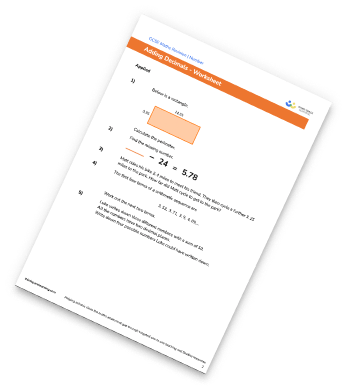Decimals Worksheets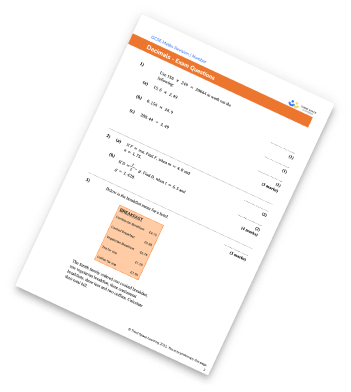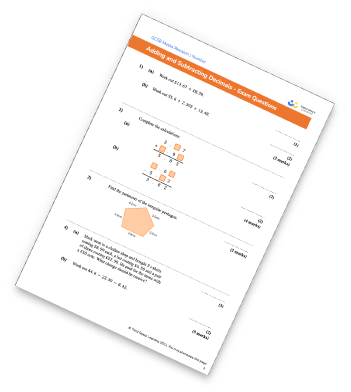Multiplying And Dividing Decimals Worksheet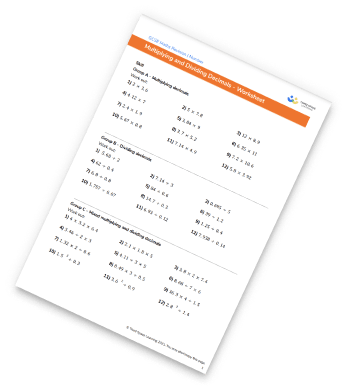## Do you have KS4 students who need more focused attention to succeed at GCSE?There will be students in your class who require individual attention to help them succeed in their maths GCSEs. In a class of 30, it’s not always easy to provide.

Help your students feel confident with exam-style questions and the strategies they’ll need to answer them correctly with our dedicated GCSE maths revision programme.

Lessons are selected to provide support where each student needs it most, and specially-trained GCSE maths tutors adapt the pitch and pace of each lesson. This ensures a personalised revision programme that raises grades and boosts confidence.

Find out more